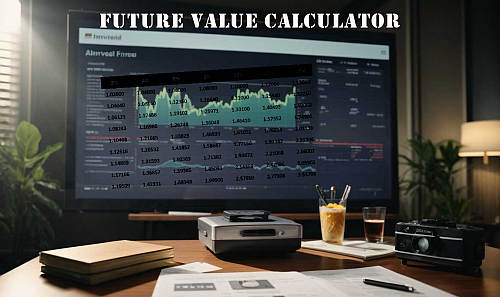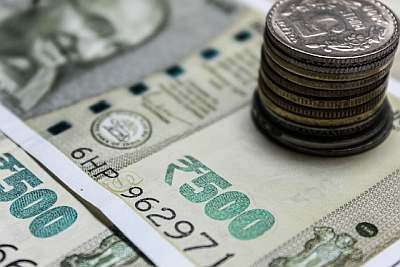# Future Value Calculator

Instructions: Compute the future value ($$FV$$) showing step-by-step, using this Future Value Calculator, by indicating the present value ($$PV$$), the interest rate ($$r$$), number of years ($$n$$) the money will be invested, and the type of compounding (yearly, bi-yearly, quarterly, monthly, weekly, daily or continuously):Present Value $$(PV)$$ =Number of Years $$(n)$$ =Interest Rate $$(r)$$ =
Compounding Period:

## Future Value Calculator

More about the this future value calculator so you can better use this solver: The future value ($$FV$$) of a certain amount of money with a certain present value ($$PV$$) depends on the number of years $$n$$ that the money will be invested, the interest rate $$r$$, the type of compounding (yearly, bi-yearly, quarterly, monthly, weekly, daily or continuously).

Let $$k$$ be the number of times the money is compounded in a year. For example, for yearly compounding we have $$k = 1$$, for bi-yearly compounding we have $$k = 2$$, for quarterly compounding we have $$k = 4$$, etc.

## How do you calculate future value?

There are several ways to compute the future value. The simplest one is to use a future value calculator like this one, or you can use a finance calculator.

If you want to do it manually, then you will have to use the present value formula. This involves knowing the formula, and applying it correct. The main advantage of our calculator is that it shows you all steps, so it is like using the formula but getting guided through the steps.

### Future Value Formula

The future value ($$FV$$) can be computed using the following formula:

$FV = PV \times \left( 1+\frac{r}{k}\right)^{ k \times n}$

For continuous compounding, we get that $$k \to \infty$$, in which case we need to use the following formula instead.

$FV = PV \times e^{r \times n}$

This calculator will compute the future value of an investment when we know the present value and the interest rates, showing all the steps. Something similar could be done with Excel using the FV formula, but Excel won't show you the steps, only the final answer.

Observe that this calculator does not consider the existence of payments. If there are periodical payments involved, you will want to check our annuity calculator , in which you are able to compute the future value with payments.## What is the easiest way to calculate future value?

Without a doubt the easiest way to compute the future value is to use a calculator. But then, there is something about calculating it manually that really can get you into a deeper understanding of how things work with present and future cash flows.

Using Excel or a financial calculator can get you really proficient at knowing what button to press, but may not help you to improve your understanding how to transition between present and future cash flows.

### Example of a Future Value: What is the future value of $100 in 2 years time? This is a typical question you may find when dealing with future values. This question is incomplete, because in order to calculate the future value of a present flow, you need the discount rate $$r$$. So, let's say that the discount rate is $$r = 10%$$. Using the FV formula, the future value of$100 in 2 years time is

$FV = PV \times \left( 1+\frac{r}{k}\right)^{ k \times n} = 100 \left( 1+10%\right)^{2} = 121$

The formula above shows k = 1 and n = 1, because the compounding is assumed to be done yearly. The result would change if the compounding was quarterly, for example.

### Future value calculator monthly

One of the most common uses of this calculator is when you need to compute a future value when the compounding occurs monthly instead of yearly. For some reason, students grasp it well when it pertains to yearly rate, but when it is monthly, some sort of block pops up.

In practicality, it is the same as yearly compouding, only that it uses an adjusted discount rate (from a nominal annual rate), and the number of compounding periods change (the number of years multiplied by 12).### Example FV calculation

Question: How much will you have in the bank in 10 years, if you put today \$10,000, when the compounding occurs monthly for a nominal annual rate of 3.5%.

Solution:

This is the information we have been provided with:

• The present value is $$PV = 10000$$, the yearly interest rate is $$r = 0.035$$. The total number of years is $$n = 10$$, and the compounding is done monthly.

Hence, the future value after 10 years (the number of compounding periods is $$12 \times 10 = 120$$ ) is calculated using the following formula:

$\begin{array}{ccl} FV & = & PV \times \left( 1+\frac{r}{k}\right)^{ k \times n} \\\\ \\\\ & = & 10000 \times \left( 1+\frac{ 0.035}{ 12}\right)^{ 12 \times 10} \\\\ \\\\ & = & 10000 \times \left( 1+ 0.0029 \right)^{ 120} = 10000 \times 1.4183 \\\\ \\\\ & = & 14183.45 \end{array}$

which means that the future value for a present value of $$PV = 10000$$, for a yearly interest rate of $$r = 0.035$$, $$n = 10$$ years, and with monthly compounding is $$FV =\text{\textdollar}14183.45$$.

### Other of our online financial calculators

You may also be interested in our present value calculator .